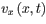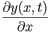# Problem: Two Velocities in a Traveling WaveWave motion is characterized by two velocities: the velocity with which the wave moves in the medium (e.g., air or a string) and the velocity of the medium (the air or the string itself).Consider a transverse wave traveling in a string. The mathematical form of the wave isy(x,t) = Asin(Kx - wt) Part AFind the velocity of propagation vp of this wave.Express the velocity of propagation in terms of some or all of the variables A, k,and w.Part BFind the y velocity  vy(x,t)  of a point on the string as a function of x and t.Express the y velocity in terms of w, A,k,x,and t.Part CWhich of the following statements about, the x component of the velocity of the string, is true?a) vx(x;t) = vpb) vx(x;t) = vy(x;t) c) vx(x;t)  has the same mathematical form as vy(x;t) but is 180° out of phase.d) vx(x;t) = 0Part DFind the slope of the string  as a function of position x and time t.Express your answer in terms of A,k,w, x,and t.

###### FREE Expert Solution

Propagation constant k:

$\overline{){\mathbf{k}}{\mathbf{=}}\frac{\mathbf{2}\mathbf{\pi }}{\mathbf{\lambda }}}$

Period, T:

$\overline{){\mathbf{T}}{\mathbf{=}}\frac{\mathbf{2}\mathbf{\pi }}{\mathbf{\omega }}}$

Part A

vp = λ/T = (2π/k)/(2π/ω) = ω/k###### Problem Details

Two Velocities in a Traveling Wave

Wave motion is characterized by two velocities: the velocity with which the wave moves in the medium (e.g., air or a string) and the velocity of the medium (the air or the string itself).

Consider a transverse wave traveling in a string. The mathematical form of the wave is

y(x,t) = Asin(Kx - wt)

Part A

Find the velocity of propagation vp of this wave.
Express the velocity of propagation in terms of some or all of the variables A, k,and w.

Part B

Find the y velocity  vy(x,t)  of a point on the string as a function of x and t.

Express the y velocity in terms of w, A,k,x,and t.

Part C

Which of the following statements about, the x component of the velocity of the string, is true?

a) vx(x;t) = vp

b) vx(x;t) = vy(x;t)

c) vx(x;t)  has the same mathematical form as vy(x;t) but is 180° out of phase.

d) vx(x;t) = 0

Part D

Find the slope of the stringas a function of position x and time t.